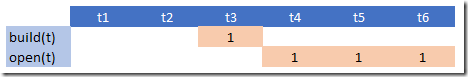## Saturday, December 31, 2016

### MIP Modeling

In an investment planning model I needed to model the following:We can build a facility during any time period. So we have:

 \begin{align}&build_t \in \{0,1\} \\ &\sum_t build_t \le 1\end{align}

The variable $$open_t\in \{0,1\}$$ indicates we have an open facility, ready to do business. A facility is considered open after it is built (we don’t close facilities during the planning horizon). To be more precise: it becomes available one period after it has been built.

There are actually a few ways to model this:

##### alternative 1

Look if the facility was built in any period $$t’<t$$:

 $open_t = \sum_{t’|t’ ##### alternative 2 Use an ‘or’ formulation:  \[open_t = build_{t-1} \textbf{ or } open_{t-1}$

This can be linearized as:

 \begin{align}&open_t \ge build_{t-1}\\ &open_t \ge open_{t-1}\\ &open_t \le build_{t-1}+open_{t-1}\end{align}

##### alternative 3

We can simplify the recursive construct:

 $open_t = build_{t-1}+open_{t-1}$

With this, we now explicitly forbid $$build_{t-1}+open_{t-1}=2$$ which was not allowed (implicitly) anyway.

The last formulation seems preferable in terms of simplicity and the number of non-zero elements needed.

As usual with lags we need to be careful what happens near the borders, in this case when $$t=t1$$. We just can fix $$open_{t1}=0$$. When using GAMS you can even skip that, as indexing with lags outside the domain returns zero. Here that means $$open_{t1} = build_{t1-1} + open_{t1-1} = 0 + 0$$. We can also verify this in the equation listing:

 ---- eqopen2  =E=  eqopen2(t1)..  open(t1) =E= 0 ; (LHS = 0)     eqopen2(t2)..  - build(t1) - open(t1) + open(t2) =E= 0 ; (LHS = 0)     eqopen2(t3)..  - build(t2) - open(t2) + open(t3) =E= 0 ; (LHS = 0)     REMAINING 3 ENTRIES SKIPPED